# Algebra 1 : How to find mode

## Example Questions

### Example Question #81 : How To Find Mode

Find the mode of the following values: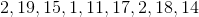Explanation:

The mode is the number that is repeated more often than the other numbers.

2, 19, 15, 1, 11, 17, 2, 18, 14

So, 2 is the mode.

### Example Question #81 : How To Find Mode

Find the mode of the following set of numbers: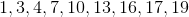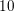All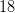NoneNone

Explanation:

The mode is the most frequent number. Since each number appears only once, their is no mode. Thus, our answer is "none".

### Example Question #83 : How To Find Mode

Find the mode of the following set of numbers: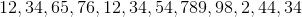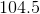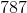Explanation:

Find the mode of the following set of numbers:Mode is simply the most repeated number in the set.

In this case, we have three 34's, so our answer must be 34. Nothing else comes close.

### Example Question #442 : Statistics And Probability

A zoo just recently took inventory of all the new animals they received from the neighboring wildlife conservatory. This was their list:

snake, kangaroo, elephant, bonobo, bonobo, snake, lemur, snake, lemur, bonobo

What is the mode of the inventory log of recently received animals?

kangaroos and elephants

elephants

snakes and bonobos

lemurs

snakes

snakes and bonobos

Explanation:

The mode is the number that appears most often in your set. In this case, because there are no numbers, we will be looking at which animal appears most often.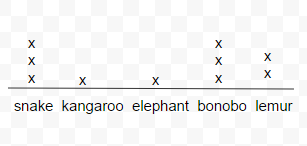This is one simple way to organize the given information. Each "X" represents each time the given animal appeared on the inventory log. The animal (in this case) with the most x's above its name appears most often and may be considered the mode. In this case, we see 3 x's for bonobos and snakes. Therefore, snakes and bonobos appear most often.

### Example Question #81 : How To Find Mode

A group of students were recording the weather for two weeks for a science project. Here was their data set: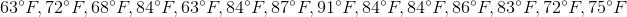What is the mode of their data set?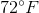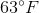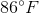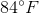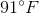Explanation:

The mode is the number that appears most often in your set. In this case we will be looking at which temperature appears most often.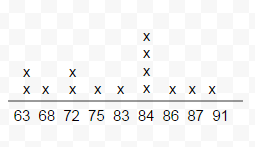This is one simple way of organizing data to indicate the mode. Each number that appeared in the data set was written down. Each "X" written above each number indicates each time it was present in the set. We see that 84 appeared most often. Therefore, 84 is the mode of the data set.

### Example Question #81 : How To Find Mode

What is the mode of this class's state exam results if the scores are the following?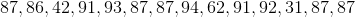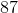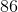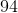Explanation:

The mode is the number that appears most often in your set. In this case we will be looking at which score appears most often.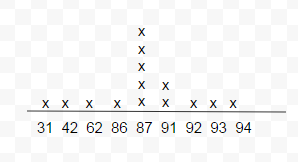This is one simple way of organizing data to indicate the mode. Each number that appeared in the data set was written down. Each "X" written above each number indicates each time it was present in the set. We see that 87 appeared most often. Therefore, 87 is the mode of the data set.

### Example Question #87 : How To Find Mode

Find the mode of the following number set: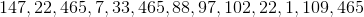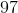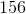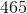Explanation:

Find the mode of the following number set:The mode is the most repeated number in a series.

To find the mode we can first arrange the terms in increasing order.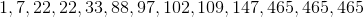From here it should be pretty clear that our answer is 465. 465 appears 3 times, the next most common number is 22, which only appears twice.

### Example Question #441 : Statistics And Probability

You take a quiz in class and are shown the results of 10 students. There were 8 questions and the score is given as 1-8. Find the mode of the following results: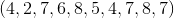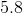Explanation:

To find the mode, you can make a number line and place an "x" for every occurance of a score or number.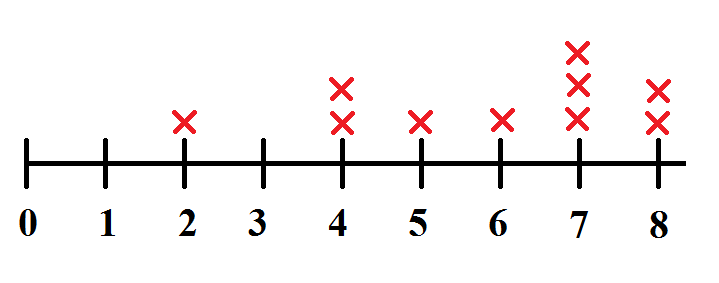The mode of a data set is the value which appears most often. In this case the mode is 7 since it occurred 3 times.

### Example Question #82 : How To Find Mode

Find the mode of the dataset: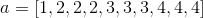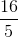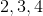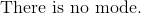Explanation:

The definition of mode is the highest frequency of numbers in the dataset.

The is one, and three of each.

Therefore, the mode must be.

The answer is:### Example Question #451 : Statistics And Probability

Find the mode in the following sets of numbers: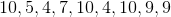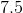Explanation:

To find the mode, we simply locate the number that occurs most often.

In this set of numbersthe number 10 occurs three times which is the most often.

### All Algebra 1 Resources